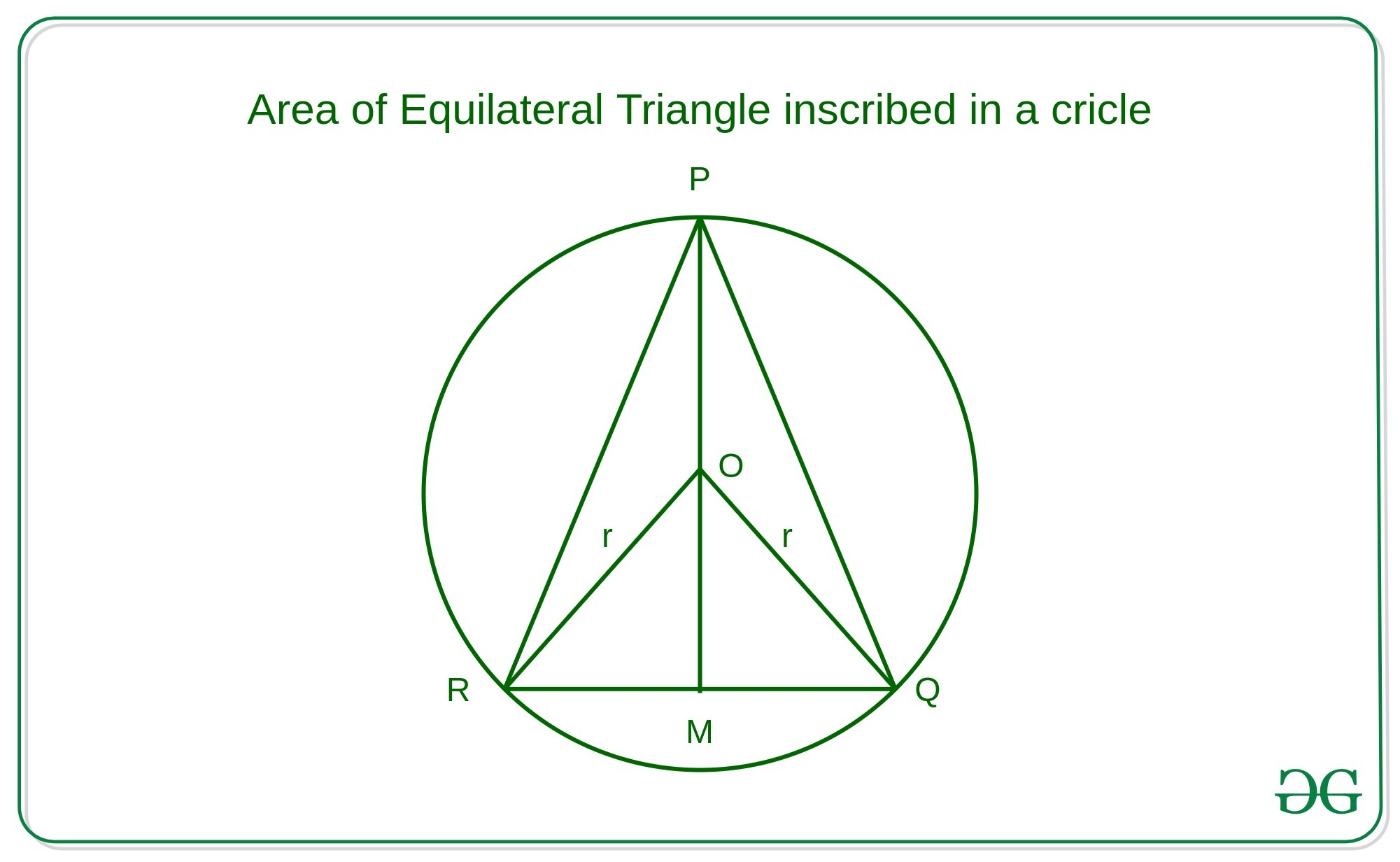GeeksforGeeks App
Open AppBrowser
Continue

# Area of Equilateral triangle inscribed in a Circle of radius R

Given an integer R which denotes the radius of a circle, the task is to find the area of an equilateral triangle inscribed in this circle.

Examples:

Input: R = 4
Output: 20.784
Explanation:
Area of equilateral triangle inscribed in a circle of radius R will be 20.784, whereas side of the triangle will be 6.928

Input: R = 7
Output: 63.651
Explanation:
Area of equilateral triangle inscribed in a circle of radius R will be 63.651, whereas side of the triangle will be 12.124

Approach: Let the above triangle shown be an equilateral triangle denoted as PQR.`Area of triangle = (1/2) * Base * Height`
• In this case, Base can be PQ, PR or QR and The height of the triangle can be PM. Hence,
`Area of Triangle = (1/2) * QR * PM`
• Now Applying sine law on the triangle ORQ
``` RQ         OR
------  = -------
sin 60    sin 30

=> RQ = OR * sin60 / sin30
=> Side of Triangle = OR * sqrt(3)

As it is clearly observed
PM = PO + OM = r + r * sin30 = (3/2) * r```
• Therefore, the Base and height of the required equilateral triangle will be:
```Base = r * sqrt(3) = r * 1.732
Height = (3/2) * r```
• Compute the area of the triangle with the help of the formulae given above.

Below is the implementation of the above approach:

## C++

 `// C++ implementation to find``// the area of the equilateral triangle``// inscribed in a circle of radius R``#include ``using` `namespace` `std;` `// Function to find the area of``// equilateral triangle inscribed``// in a circle of radius R``double` `area(``int` `R) {``     ` `     ``// Base and Height of``    ``// equilateral triangle``    ``double` `base = 1.732 * R;``    ``double` `height = (1.5) * R;``     ` `            ``// Area using Base and Height``    ``double` `area = 0.5 * base * height;``    ``return` `area;``}` `// Driver Code``int` `main()``{``    ``int` `R = 7;``    ``cout<<(area(R));``    ``return` `0;``}` `// This code is contributed by 29AjayKumar`

## Java

 `// Java implementation to find``// the area of the equilateral triangle``// inscribed in a circle of radius R``class` `GFG``{``    ``// Function to find the area of``    ``// equilateral triangle inscribed``    ``// in a circle of radius R``    ``static` `double` `area(``int` `R) {``        ` `                ``// Base and Height of``        ``// equilateral triangle``        ``double` `base = ``1.732` `* R;``        ``double` `height = (``1.5``) * R;``        ` `                ``// Area using Base and Height``        ``double` `area = ``0.5` `* base * height;``        ``return` `area;``    ``}` `    ``// Driver code``    ``public` `static` `void` `main(String[] args) {``        ``int` `R = ``7``;``        ``System.out.println(area(R));` `    ``}``}` `// This code is contributed by 29AjayKumar`

## Python3

 `# Python 3 implementation to find``# the area of the equilateral triangle``# inscribed in a circle of radius R` `# Function to find the area of``# equilateral triangle inscribed``# in a circle of radius R``def` `area(R):``    ``# Base and Height of``    ``# equilateral triangle``    ``base ``=` `1.732` `*` `R``    ``height ``=` `( ``3` `/` `2` `) ``*` `R``    ` `    ``# Area using Base and Height``    ``area ``=` `(( ``1` `/` `2` `) ``*` `base ``*` `height )``    ``return` `area``    ` `# Driver Code``if` `__name__``=``=``'__main__'``:``    ``R ``=` `7``    ``print``(area(R))`

## C#

 `// C# implementation to find``// the area of the equilateral triangle``// inscribed in a circle of radius R``using` `System;` `class` `GFG``{``    ``// Function to find the area of``    ``// equilateral triangle inscribed``    ``// in a circle of radius R``    ``static` `double` `area(``int` `R)``    ``{``        ` `        ``// Base and Height of``        ``// equilateral triangle``        ``double` `Base = 1.732 * R;``        ``double` `height = (1.5) * R;``        ` `        ``// Area using Base and Height``        ``double` `area = 0.5 * Base * height;``        ``return` `area;``    ``}` `    ``// Driver code``    ``public` `static` `void` `Main(String[] args)``    ``{``        ``int` `R = 7;``        ``Console.WriteLine(area(R));``    ``}``}` `// This code is contributed by 29AjayKumar`

## Javascript

 ``

Output:

`63.651`

Time complexity : O(1)
Auxiliary Space : O(1)

My Personal Notes arrow_drop_up